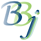# SETTERM Verb - Reset Terminal Alias (BBj)

## Syntax

SETTERM alias{,MODE="NOALIAS"}{,ERR=stmt}

## Description

The SETTERM verb resets the terminal alias, FID(0). BBj takes the optional ERR= branch if the specified alias does not appear as a terminal alias in the config.bbx file.

SETTERM can be used to force FID(0) to a selected value if required by the application, typically if the application runs in a browser.

 Parameter Description alias New value of FID(0). This value must always start with "T". MODE="NOALIAS" By default, SETTERM verifies that the new FID(0) value is a terminal alias defined in the config.bbx file. Specifying MODE="NOALIAS" causes it to skip this step. ERR=lineref Branch taken if an error occurs during execution.

## Example 1

This example assumes that the terminal aliases in the config.bbx file have been defined with USERID=XXX modes to associate users with terminal ids. For example:

 ALIAS T0 SYSWINDOW "" USERID=ABC ALIAS T1 SYSWINDOW "" USERID=DEF ALIAS T2 SYSWINDOW "" USERID=GHI ALIAS T3 SYSWINDOW "" USERID=JKL ALIAS T4 SYSWINDOW "" USERID=MNO

The application should start with a login step that prompts for a user id, then resets FID(0) to the corresponding terminal id. See the example below:

 0010 REM ' Login 0020 PRINT 'TITLE'("Login") 0030 DIM TSK\$:"TYPE:U(1),*:U(1),ALIAS:C(1*=0),*:C(1*=0),*:C(1*=0),MODES:C(1*=0)" 0040 USERID: 0050 INPUT 'CS',@(10,10),"Userid: ",USERID\$ 0060 IF CTL=4 THEN RELEASE 0070 LET TSK=0,TEMP\$="USERID="+CVS(USERID\$,7)+"," 0080 LOOP: LET TSK\$=TSK(TSK,ERR=USERID) 0090 IF POS(TEMP\$=CVS(TSK.MODES\$,7)+",")=0 THEN LET TSK=TSK+1; GOTO LOOP 0100 SETTERM TSK.ALIAS\$; REM ' Reset FID(0) 0110 PRINT 'TITLE'("User: "+USERID\$+" ("+FID(0)+")") 0120 ESCAPE